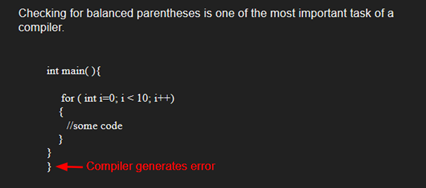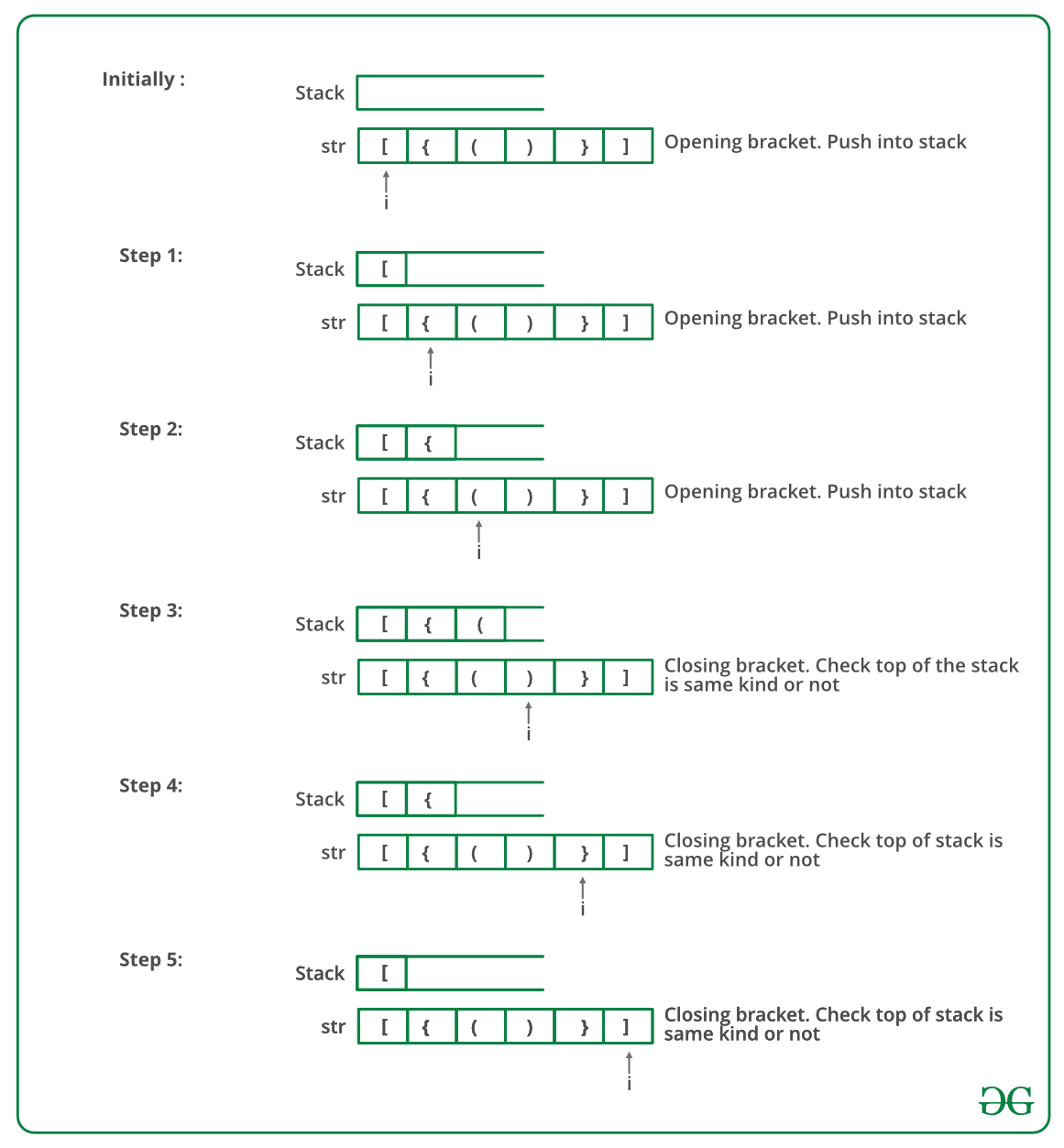Related Articles

# Check for Balanced Brackets in an expression (well-formedness) using Stack

• Difficulty Level : Easy
• Last Updated : 06 Jun, 2021

Given an expression string exp, write a program to examine whether the pairs and the orders of “{“, “}”, “(“, “)”, “[“, “]” are correct in exp.

Example

Input: exp = “[()]{}{[()()]()}”
Output: Balanced

Input: exp = “[(])”
Output: Not BalancedAlgorithm:

• Declare a character stack S.
• Now traverse the expression string exp.
1. If the current character is a starting bracket (‘(‘ or ‘{‘ or ‘[‘) then push it to stack.
2. If the current character is a closing bracket (‘)’ or ‘}’ or ‘]’) then pop from stack and if the popped character is the matching starting bracket then fine else brackets are not balanced.
• After complete traversal, if there is some starting bracket left in stack then “not balanced”

Below image is a dry run of the above approach:Below is the implementation of the above approach:

## C++

 `// CPP program to check for balanced brackets.``#include ``using` `namespace` `std;` `// function to check if brackets are balanced``bool` `areBracketsBalanced(string expr)``{ ``    ``stack<``char``> s;``    ``char` `x;` `    ``// Traversing the Expression``    ``for` `(``int` `i = 0; i < expr.length(); i++)``    ``{``        ``if` `(expr[i] == ``'('` `|| expr[i] == ``'['``            ``|| expr[i] == ``'{'``)``        ``{``            ``// Push the element in the stack``            ``s.push(expr[i]);``            ``continue``;``        ``}` `        ``// IF current current character is not opening``        ``// bracket, then it must be closing. So stack``        ``// cannot be empty at this point.``        ``if` `(s.empty())``            ``return` `false``;` `        ``switch` `(expr[i]) {``        ``case` `')'``:``            ` `            ``// Store the top element in a``            ``x = s.top();``            ``s.pop();``            ``if` `(x == ``'{'` `|| x == ``'['``)``                ``return` `false``;``            ``break``;` `        ``case` `'}'``:` `            ``// Store the top element in b``            ``x = s.top();``            ``s.pop();``            ``if` `(x == ``'('` `|| x == ``'['``)``                ``return` `false``;``            ``break``;` `        ``case` `']'``:` `            ``// Store the top element in c``            ``x = s.top();``            ``s.pop();``            ``if` `(x == ``'('` `|| x == ``'{'``)``                ``return` `false``;``            ``break``;``        ``}``    ``}` `    ``// Check Empty Stack``    ``return` `(s.empty());``}` `// Driver code``int` `main()``{``    ``string expr = ``"{()}[]"``;` `    ``// Function call``    ``if` `(areBracketsBalanced(expr))``        ``cout << ``"Balanced"``;``    ``else``        ``cout << ``"Not Balanced"``;``    ``return` `0;``}`

## C

 `#include ``#include ``#define bool int` `// structure of a stack node``struct` `sNode {``    ``char` `data;``    ``struct` `sNode* next;``};` `// Function to push an item to stack``void` `push(``struct` `sNode** top_ref, ``int` `new_data);` `// Function to pop an item from stack``int` `pop(``struct` `sNode** top_ref);` `// Returns 1 if character1 and character2 are matching left``// and right Brackets``bool` `isMatchingPair(``char` `character1, ``char` `character2)``{``    ``if` `(character1 == ``'('` `&& character2 == ``')'``)``        ``return` `1;``    ``else` `if` `(character1 == ``'{'` `&& character2 == ``'}'``)``        ``return` `1;``    ``else` `if` `(character1 == ``'['` `&& character2 == ``']'``)``        ``return` `1;``    ``else``        ``return` `0;``}` `// Return 1 if expression has balanced Brackets``bool` `areBracketsBalanced(``char` `exp``[])``{``    ``int` `i = 0;` `    ``// Declare an empty character stack``    ``struct` `sNode* stack = NULL;` `    ``// Traverse the given expression to check matching``    ``// brackets``    ``while` `(``exp``[i])``    ``{``        ``// If the exp[i] is a starting bracket then push``        ``// it``        ``if` `(``exp``[i] == ``'{'` `|| ``exp``[i] == ``'('` `|| ``exp``[i] == ``'['``)``            ``push(&stack, ``exp``[i]);` `        ``// If exp[i] is an ending bracket then pop from``        ``// stack and check if the popped bracket is a``        ``// matching pair*/``        ``if` `(``exp``[i] == ``'}'` `|| ``exp``[i] == ``')'``            ``|| ``exp``[i] == ``']'``) {` `            ``// If we see an ending bracket without a pair``            ``// then return false``            ``if` `(stack == NULL)``                ``return` `0;` `            ``// Pop the top element from stack, if it is not``            ``// a pair bracket of character then there is a``            ``// mismatch.``            ``// his happens for expressions like {(})``            ``else` `if` `(!isMatchingPair(pop(&stack), ``exp``[i]))``                ``return` `0;``        ``}``        ``i++;``    ``}` `    ``// If there is something left in expression then there``    ``// is a starting bracket without a closing``    ``// bracket``    ``if` `(stack == NULL)``        ``return` `1; ``// balanced``    ``else``        ``return` `0; ``// not balanced``}` `// Driver code``int` `main()``{``    ``char` `exp`` = ``"{()}[]"``;` `    ``// Function call``    ``if` `(areBracketsBalanced(``exp``))``        ``printf``(``"Balanced \n"``);``    ``else``        ``printf``(``"Not Balanced \n"``);``    ``return` `0;``}` `// Function to push an item to stack``void` `push(``struct` `sNode** top_ref, ``int` `new_data)``{``    ``// allocate node``    ``struct` `sNode* new_node``        ``= (``struct` `sNode*)``malloc``(``sizeof``(``struct` `sNode));` `    ``if` `(new_node == NULL) {``        ``printf``(``"Stack overflow n"``);``        ``getchar``();``        ``exit``(0);``    ``}` `    ``// put in the data``    ``new_node->data = new_data;` `    ``// link the old list off the new node``    ``new_node->next = (*top_ref);` `    ``// move the head to point to the new node``    ``(*top_ref) = new_node;``}` `// Function to pop an item from stack``int` `pop(``struct` `sNode** top_ref)``{``    ``char` `res;``    ``struct` `sNode* top;` `    ``// If stack is empty then error``    ``if` `(*top_ref == NULL) {``        ``printf``(``"Stack overflow n"``);``        ``getchar``();``        ``exit``(0);``    ``}``    ``else` `{``        ``top = *top_ref;``        ``res = top->data;``        ``*top_ref = top->next;``        ``free``(top);``        ``return` `res;``    ``}``}`

## Java

 `// Java program for checking``// balanced brackets``import` `java.util.*;` `public` `class` `BalancedBrackets {` `    ``// function to check if brackets are balanced``    ``static` `boolean` `areBracketsBalanced(String expr)``    ``{``        ``// Using ArrayDeque is faster than using Stack class``        ``Deque stack``            ``= ``new` `ArrayDeque();` `        ``// Traversing the Expression``        ``for` `(``int` `i = ``0``; i < expr.length(); i++)``        ``{``            ``char` `x = expr.charAt(i);` `            ``if` `(x == ``'('` `|| x == ``'['` `|| x == ``'{'``)``            ``{``                ``// Push the element in the stack``                ``stack.push(x);``                ``continue``;``            ``}` `            ``// If current character is not opening``            ``// bracket, then it must be closing. So stack``            ``// cannot be empty at this point.``            ``if` `(stack.isEmpty())``                ``return` `false``;``            ``char` `check;``            ``switch` `(x) {``            ``case` `')'``:``                ``check = stack.pop();``                ``if` `(check == ``'{'` `|| check == ``'['``)``                    ``return` `false``;``                ``break``;` `            ``case` `'}'``:``                ``check = stack.pop();``                ``if` `(check == ``'('` `|| check == ``'['``)``                    ``return` `false``;``                ``break``;` `            ``case` `']'``:``                ``check = stack.pop();``                ``if` `(check == ``'('` `|| check == ``'{'``)``                    ``return` `false``;``                ``break``;``            ``}``        ``}` `        ``// Check Empty Stack``        ``return` `(stack.isEmpty());``    ``}` `    ``// Driver code``    ``public` `static` `void` `main(String[] args)``    ``{``        ``String expr = ``"([{}])"``;` `        ``// Function call``        ``if` `(areBracketsBalanced(expr))``            ``System.out.println(``"Balanced "``);``        ``else``            ``System.out.println(``"Not Balanced "``);``    ``}``}`

## Python3

 `# Python3 program to check for``# balanced brackets.` `# function to check if``# brackets are balanced`  `def` `areBracketsBalanced(expr):``    ``stack ``=` `[]` `    ``# Traversing the Expression``    ``for` `char ``in` `expr:``        ``if` `char ``in` `[``"("``, ``"{"``, ``"["``]:` `            ``# Push the element in the stack``            ``stack.append(char)``        ``else``:` `            ``# IF current character is not opening``            ``# bracket, then it must be closing.``            ``# So stack cannot be empty at this point.``            ``if` `not` `stack:``                ``return` `False``            ``current_char ``=` `stack.pop()``            ``if` `current_char ``=``=` `'('``:``                ``if` `char !``=` `")"``:``                    ``return` `False``            ``if` `current_char ``=``=` `'{'``:``                ``if` `char !``=` `"}"``:``                    ``return` `False``            ``if` `current_char ``=``=` `'['``:``                ``if` `char !``=` `"]"``:``                    ``return` `False` `    ``# Check Empty Stack``    ``if` `stack:``        ``return` `False``    ``return` `True`  `# Driver Code``if` `__name__ ``=``=` `"__main__"``:``    ``expr ``=` `"{()}[]"` `    ``# Function call``    ``if` `areBracketsBalanced(expr):``        ``print``(``"Balanced"``)``    ``else``:``        ``print``(``"Not Balanced"``)` `# This code is contributed by AnkitRai01 and improved``# by Raju Pitta`

## C#

 `// C# program for checking``// balanced Brackets``using` `System;``using` `System.Collections.Generic;` `public` `class` `BalancedBrackets {``    ``public` `class` `stack {``        ``public` `int` `top = -1;``        ``public` `char``[] items = ``new` `char``;` `        ``public` `void` `push(``char` `x)``        ``{``            ``if` `(top == 99)``            ``{``                ``Console.WriteLine(``"Stack full"``);``            ``}``            ``else` `{``                ``items[++top] = x;``            ``}``        ``}` `        ``char` `pop()``        ``{``            ``if` `(top == -1)``            ``{``                ``Console.WriteLine(``"Underflow error"``);``                ``return` `'\0'``;``            ``}``            ``else``            ``{``                ``char` `element = items[top];``                ``top--;``                ``return` `element;``            ``}``        ``}` `        ``Boolean isEmpty()``        ``{``            ``return` `(top == -1) ? ``true` `: ``false``;``        ``}``    ``}` `    ``// Returns true if character1 and character2``    ``// are matching left and right brackets */``    ``static` `Boolean isMatchingPair(``char` `character1,``                                  ``char` `character2)``    ``{``        ``if` `(character1 == ``'('` `&& character2 == ``')'``)``            ``return` `true``;``        ``else` `if` `(character1 == ``'{'` `&& character2 == ``'}'``)``            ``return` `true``;``        ``else` `if` `(character1 == ``'['` `&& character2 == ``']'``)``            ``return` `true``;``        ``else``            ``return` `false``;``    ``}` `    ``// Return true if expression has balanced``    ``// Brackets``    ``static` `Boolean areBracketsBalanced(``char``[] exp)``    ``{``        ``// Declare an empty character stack */``        ``Stack<``char``> st = ``new` `Stack<``char``>();` `        ``// Traverse the given expression to``        ``//   check matching brackets``        ``for` `(``int` `i = 0; i < exp.Length; i++)``        ``{``            ``// If the exp[i] is a starting``            ``// bracket then push it``            ``if` `(exp[i] == ``'{'` `|| exp[i] == ``'('``                ``|| exp[i] == ``'['``)``                ``st.Push(exp[i]);` `            ``//  If exp[i] is an ending bracket``            ``//  then pop from stack and check if the``            ``//   popped bracket is a matching pair``            ``if` `(exp[i] == ``'}'` `|| exp[i] == ``')'``                ``|| exp[i] == ``']'``) {` `                ``// If we see an ending bracket without``                ``//   a pair then return false``                ``if` `(st.Count == 0)``                ``{``                    ``return` `false``;``                ``}` `                ``// Pop the top element from stack, if``                ``// it is not a pair brackets of``                ``// character then there is a mismatch. This``                ``// happens for expressions like {(})``                ``else` `if` `(!isMatchingPair(st.Pop(),``                                         ``exp[i])) {``                    ``return` `false``;``                ``}``            ``}``        ``}` `        ``// If there is something left in expression``        ``// then there is a starting bracket without``        ``// a closing bracket` `        ``if` `(st.Count == 0)``            ``return` `true``; ``// balanced``        ``else``        ``{``            ``// not balanced``            ``return` `false``;``        ``}``    ``}` `    ``// Driver code``    ``public` `static` `void` `Main(String[] args)``    ``{``        ``char``[] exp = { ``'{'``, ``'('``, ``')'``, ``'}'``, ``'['``, ``']'` `};` `        ``// Function call``        ``if` `(areBracketsBalanced(exp))``            ``Console.WriteLine(``"Balanced "``);``        ``else``            ``Console.WriteLine(``"Not Balanced "``);``    ``}``}` `// This code is contributed by 29AjayKumar`

## Javascript

 ``
Output
`Balanced`

Time Complexity: O(n)
Auxiliary Space: O(n) for stack.

Please write comments if you find any bug in above codes/algorithms, or find other ways to solve the same problem

Attention reader! Don’t stop learning now. Get hold of all the important DSA concepts with the DSA Self Paced Course at a student-friendly price and become industry ready.  To complete your preparation from learning a language to DS Algo and many more,  please refer Complete Interview Preparation Course.

In case you wish to attend live classes with experts, please refer DSA Live Classes for Working Professionals and Competitive Programming Live for Students.

My Personal Notes arrow_drop_up